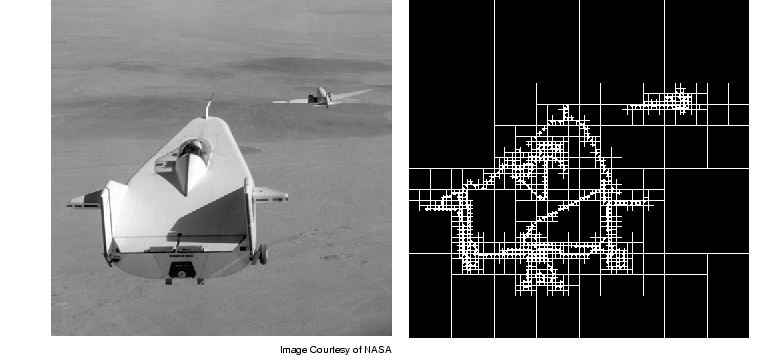Image Processing Toolbox User's GuideQuadtree decomposition is an analysis technique that involves subdividing an image into blocks that are more homogeneous than the image itself. This technique reveals information about the structure of the image. It is also useful as the first step in adaptive compression algorithms.

You can perform quadtree decomposition using the `qtdecomp` function. This function works by dividing a square image into four equal-sized square blocks, and then testing each block to see if it meets some criterion of homogeneity (e.g., if all the pixels in the block are within a specific dynamic range). If a block meets the criterion, it is not divided any further. If it does not meet the criterion, it is subdivided again into four blocks, and the test criterion is applied to those blocks. This process is repeated iteratively until each block meets the criterion. The result might have blocks of several different sizes.

To illustrate, this example performs quadtree decomposition on a 512-by-512 grayscale image. For an interactive demonstration of quadtree decomposition, run the demo `qtdemo`.

1. Read in the grayscale image.
• ```I = imread('liftingbody.png');
```
2. Specify the test criteria used to determine the homogeneity of each block in the decomposition. For example, the criterion might be this threshold calculation.
• ```max(block(:)) - min(block(:)) <= 0.2
```
1. You can also supply `qtdecomp` with a function (rather than a threshold value) for deciding whether to split blocks; for example, you might base the decision on the variance of the block. See the reference page for `qtdecomp` for more information.

1. Perform this quadtree decomposition by calling the `qtdecomp` function, specifying the image and the threshold value as arguments.
• ```S = qtdecomp(I,0.27)
```
1. You specify the threshold as a value between 0 and 1, regardless of the class of `I`. If `I` is `uint8`, `qtdecomp` multiplies the threshold value by 255 to determine the actual threshold to use. If `I` is `uint16`, `qtdecomp` multiplies the threshold value by 65535.

`qtdecomp` first divides the image into four 256-by-256 blocks and applies the test criterion to each block. If a block does not meet the criterion, `qtdecomp` subdivides it and applies the test criterion to each block. `qtdecomp` continues to subdivide blocks until all blocks meet the criterion. Blocks can be as small as 1-by-1, unless you specify otherwise.

`qtdecomp` returns `S` as a sparse matrix, the same size as `I`. The nonzero elements of `S` represent the upper left corners of the blocks; the value of each nonzero element indicates the block size.

The following figure shows the original image and a representation of its quadtree decomposition. (To see how this representation was created, see the example on the `qtdecomp` reference page.) Each black square represents a homogeneous block, and the white lines represent the boundaries between blocks. Notice how the blocks are smaller in areas corresponding to large changes in intensity in the image.Image and a Representation of Its Quadtree DecompositionDetecting Lines Using the Hough Transform Analyzing the Texture of an Image